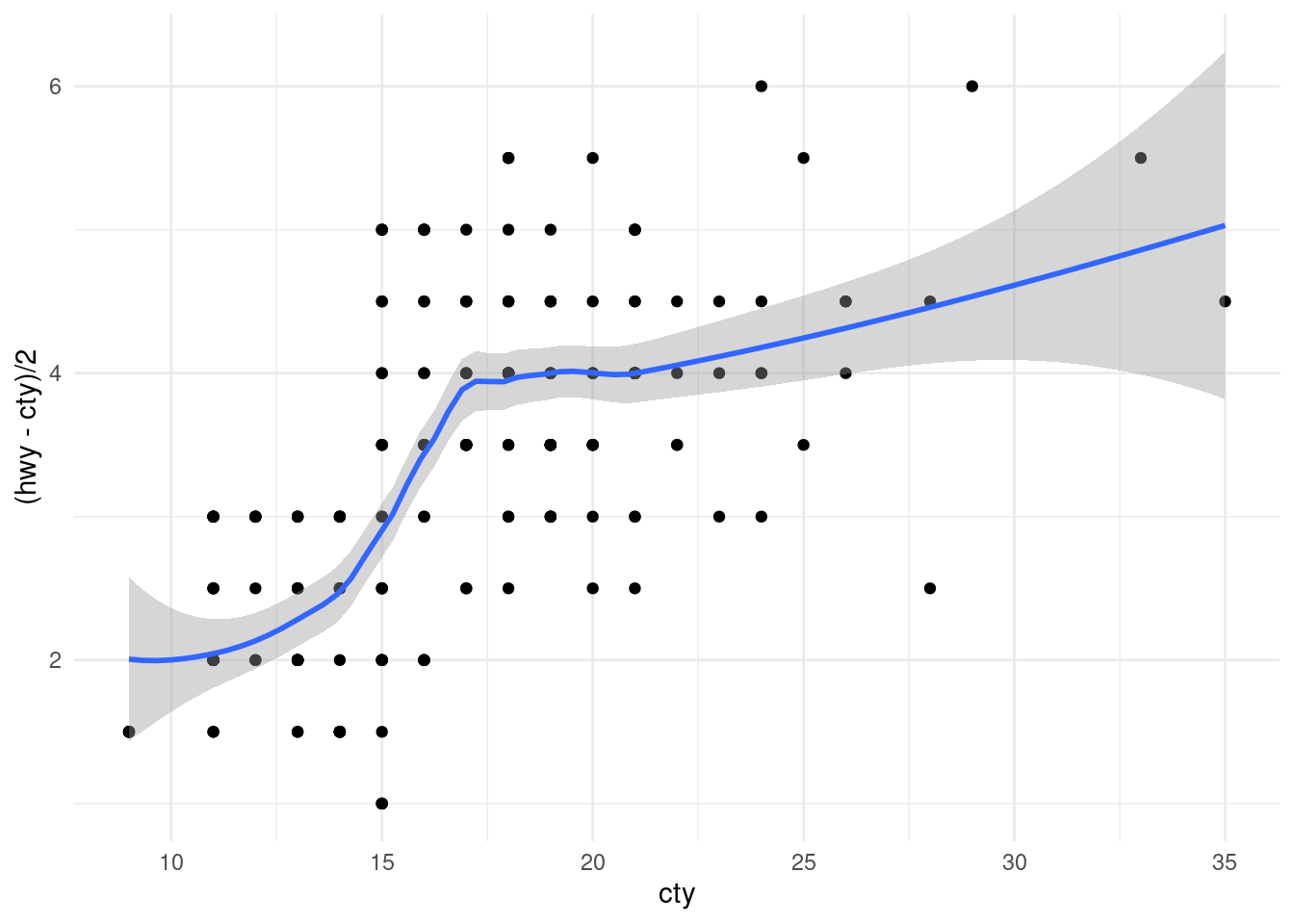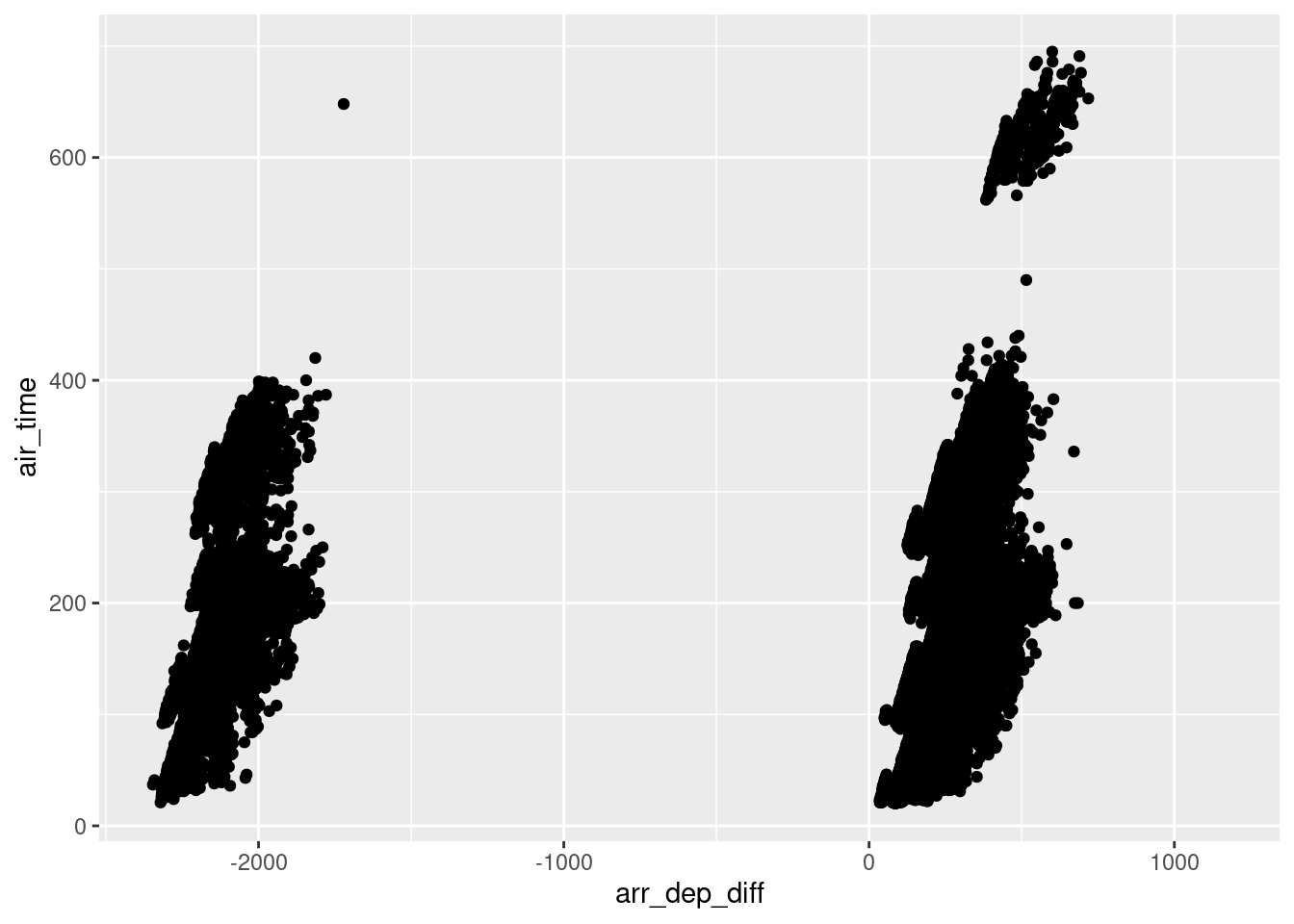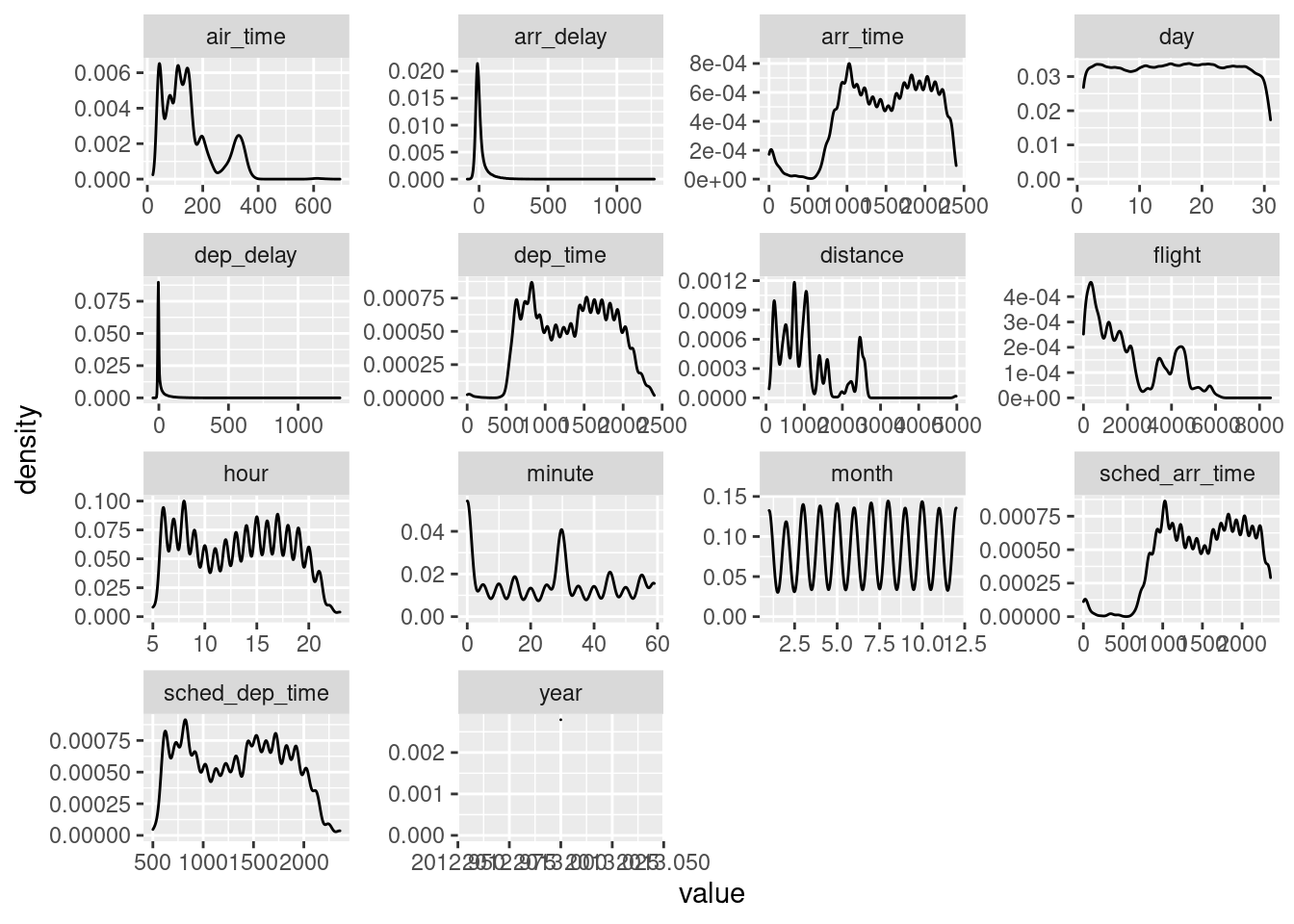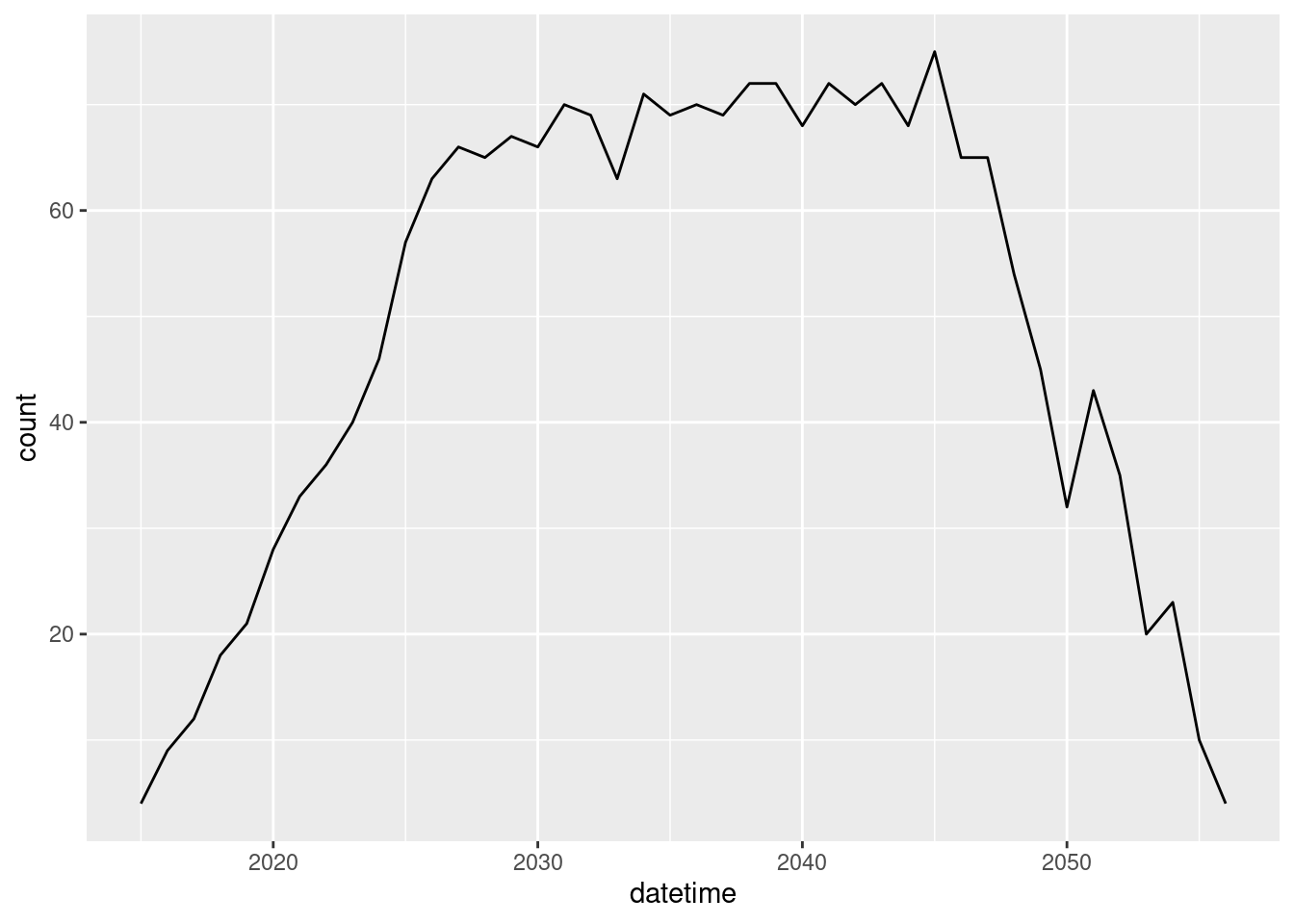Computer “bugs” enter code because people have a hard time communicating with computers. If we were giving instructions to a person, then they would use their best judgment to correct for any small errors or ambiguities. Computers, on the other hand, follow instructions exactly.

Sometimes these bugs will be bad enough that a program just doesn’t know what to do and won’t run. In these cases, the program will quit and will display an error message (also called “throwing” an error).

More dangerously, a program will run but it won’t quite be doing what we want or expect it to do.

The process of finding bugs is called quality assurance or testing, and the process of figuring out what part of the code is causing the bug and fixing it is called “deubgging”.

In this quick tutorial, I’m going to teach you a few basic principles of debugging and writing code that makes bugs easier to find. I’ll be using the `tidyverse` in R, which is designed to be readable and easier to understand.

## Syntax bugs

Some of the most common bugs for new programmers are syntax bugs. Programming syntax is confusing and complicated, and just like learning a new human langauge it takes practice before it becomes natural.

The syntax errors that I find most common are: missing commas, missing parentheses/brackets, and problems with nested parentheses.

### Commas

See if you can spot the problem with this code:

``````values <- 1:50
sample(values,
size = 20
replace = TRUE
)``````

When we run it, R gives us a somewhat confusing message - that there is an “unexpected symbol”. It does give us a clue of where to look for the problem, but the issue is not an extra symbol, it’s actually a missing symbol - we need a comma after setting the `size` parameter to `20`.

### Missing parentheses

Find the bug:

``````mpg %>%
ggplot() + geom_point(aes(y = hwy, x = displ) + theme_minimal()``````

Here, R is more helpful and identifies this as an incomplete expression.

### Nested parentheses problems

Other than just missing parentheses, nested parentheses can cause some other bugs. This code is supposed to plot half of the difference between highway and city mileage on the y axis.

``````mpg %>%
ggplot(aes(y = (hwy - cty)/2), x = cty) + geom_point() + theme_minimal() + geom_smooth()``````

This time, the error message is only helpful with some detective work. It is telling us that `stat_smooth` which is invisibly called by `geom_smooth` is missing the `x` aesthetic. This gives us a hint that the problem has to do with how the `x` gets set. Indeed, if you look carefully, there is a misplaced ending parenthesis, and `x` is outside of the `aes()`.

Here’s a fixed version:

``````mpg %>%
ggplot(aes(y = (hwy - cty)/2, x = cty)) + geom_point() + theme_minimal() + geom_smooth()``````
``## `geom_smooth()` using method = 'loess' and formula 'y ~ x'``One strategy for finding and avoiding these problems is to put arguments on new lines. Here is the same bug, this time with better spacing.

If you are using RStudio, then pressing `Enter` will create a new line and will indent the next line to the right place - if it indents it somewhere else (as below) then this can help to find bugs.

``````mpg %>%
ggplot(
aes(
y = (hwy - cty)/2),
x = cty) +
geom_point() +
theme_minimal() +
geom_smooth()``````

If we had written it correctly, then it would have indented like this.

``````mpg %>%
ggplot(
aes(
y = (hwy - cty)/2,
x = cty
)
) +
geom_point() +
theme_minimal() +
geom_smooth()``````
``## `geom_smooth()` using method = 'loess' and formula 'y ~ x'``Note how when you put your cursor next to any parenthesis, it shows the matching parenthesis. This is also a good strategy for finding bugs.

## Data Bugs

The other major type of bugs that I want to touch on I’m calling “Data Bugs”. These are bugs where the data that you have is not what you think it is. This can happen in a lot of ways. Here are a few of the most common.

### Misunderstanding of variables

Sometimes we may be using a dataset that we just don’t understand. In R for Data Science Chapter 5 the authors use the `nycflights` data set. When introducing `mutate`, we might assume that `air_time` for a flight is equal to `arr_time` - `dep_time`, but it isn’t.

``````flights %>%
mutate(arr_dep_diff = arr_time - dep_time) %>%
ggplot(aes(y = air_time, x = arr_dep_diff)) +
geom_point()``````
``## Warning: Removed 9430 rows containing missing values (geom_point).``The first problem we see is that sometimes arrival - departure is negative! How is that possible? Well, `arr_time` and `dep_time` are times (represented as integers), so if a flight could leave at 11:00 pm (2300) and arrive at 3 am (300), leaving a negative number.

More than that, however, there are some other issues. Most obviously, you can’t subtract times and get minutes. 5:30 - 3:30 is 120 minutes, but if we naively subtract 530 - 330 we get 200. In other words, the format of the data does not match the operation that we want.

Also, there are time zones to consider - the `arr_time` and `dep_time` are in local times.

Finally, `air_time` may just include the time actually in the air, while `dep_time` and `arr_time` might include taxiing. If we don’t fix each of these issues, then we could make errors in interpretation.

There are a few strategies for avoiding these kinds of bugs. The first is to read the documentation about the variables, if it exists, to figure out what they are measuring. The second is to look at the data - look at the data frames, plot the histograms for each variable, etc.

Some tools for doing this:

``head(flights)``
``````## # A tibble: 6 x 19
##    year month   day dep_time sched_dep_time dep_delay arr_time sched_arr_time
##   <int> <int> <int>    <int>          <int>     <dbl>    <int>          <int>
## 1  2013     1     1      517            515         2      830            819
## 2  2013     1     1      533            529         4      850            830
## 3  2013     1     1      542            540         2      923            850
## 4  2013     1     1      544            545        -1     1004           1022
## 5  2013     1     1      554            600        -6      812            837
## 6  2013     1     1      554            558        -4      740            728
## # … with 11 more variables: arr_delay <dbl>, carrier <chr>, flight <int>,
## #   tailnum <chr>, origin <chr>, dest <chr>, air_time <dbl>, distance <dbl>,
## #   hour <dbl>, minute <dbl>, time_hour <dttm>``````
``summary(flights)``
``````##       year          month             day           dep_time    sched_dep_time
##  Min.   :2013   Min.   : 1.000   Min.   : 1.00   Min.   :   1   Min.   : 106
##  1st Qu.:2013   1st Qu.: 4.000   1st Qu.: 8.00   1st Qu.: 907   1st Qu.: 906
##  Median :2013   Median : 7.000   Median :16.00   Median :1401   Median :1359
##  Mean   :2013   Mean   : 6.549   Mean   :15.71   Mean   :1349   Mean   :1344
##  3rd Qu.:2013   3rd Qu.:10.000   3rd Qu.:23.00   3rd Qu.:1744   3rd Qu.:1729
##  Max.   :2013   Max.   :12.000   Max.   :31.00   Max.   :2400   Max.   :2359
##                                                  NA's   :8255
##    dep_delay          arr_time    sched_arr_time   arr_delay
##  Min.   : -43.00   Min.   :   1   Min.   :   1   Min.   : -86.000
##  1st Qu.:  -5.00   1st Qu.:1104   1st Qu.:1124   1st Qu.: -17.000
##  Median :  -2.00   Median :1535   Median :1556   Median :  -5.000
##  Mean   :  12.64   Mean   :1502   Mean   :1536   Mean   :   6.895
##  3rd Qu.:  11.00   3rd Qu.:1940   3rd Qu.:1945   3rd Qu.:  14.000
##  Max.   :1301.00   Max.   :2400   Max.   :2359   Max.   :1272.000
##  NA's   :8255      NA's   :8713                  NA's   :9430
##    carrier              flight       tailnum             origin
##  Length:336776      Min.   :   1   Length:336776      Length:336776
##  Class :character   1st Qu.: 553   Class :character   Class :character
##  Mode  :character   Median :1496   Mode  :character   Mode  :character
##                     Mean   :1972
##                     3rd Qu.:3465
##                     Max.   :8500
##
##      dest              air_time        distance         hour
##  Length:336776      Min.   : 20.0   Min.   :  17   Min.   : 1.00
##  Class :character   1st Qu.: 82.0   1st Qu.: 502   1st Qu.: 9.00
##  Mode  :character   Median :129.0   Median : 872   Median :13.00
##                     Mean   :150.7   Mean   :1040   Mean   :13.18
##                     3rd Qu.:192.0   3rd Qu.:1389   3rd Qu.:17.00
##                     Max.   :695.0   Max.   :4983   Max.   :23.00
##                     NA's   :9430
##      minute        time_hour
##  Min.   : 0.00   Min.   :2013-01-01 05:00:00
##  1st Qu.: 8.00   1st Qu.:2013-04-04 13:00:00
##  Median :29.00   Median :2013-07-03 10:00:00
##  Mean   :26.23   Mean   :2013-07-03 05:22:54
##  3rd Qu.:44.00   3rd Qu.:2013-10-01 07:00:00
##  Max.   :59.00   Max.   :2013-12-31 23:00:00
## ``````
``````flights %>%
na.omit() %>%
keep(is.numeric) %>%
gather() %>%
ggplot(aes(value)) +
facet_wrap(~ key, scales = "free") +
geom_density()``````### Bad transformations

The second big class of bugs is where we are doing something to the data, but not doing what we think we are. Sometimes this is a simple as a typo.

For example, say we wanted to plot the number of flights per day to Indianapolis.

``````flights %>%
filter(dest == "IND") %>% # Just get IND flights
mutate(datetime = year + month + day) %>% # Calculate a datetime by combining year, month, and day
group_by(datetime) %>% # Group flights by datetime
mutate(count = n()) %>% # Count the number of flights each day
ggplot(aes(y = count, x = datetime)) +
geom_line()``````This seems just fine until we look at the plot! Why does this go until 2060?

Aha! We were trying to combine year, month, and day into a datetime, but R just treated them as numbers and added them together.

The best way to do this is to actually use something like `lubridate` to make this into a `date` object.

``library(lubridate)``
``````##
## Attaching package: 'lubridate'``````
``````## The following object is masked from 'package:base':
##
##     date``````
``````flights %>%
filter(dest == "IND") %>% # Just get IND flights
mutate(datetime = make_date(year, month, day)) %>% # Calculate a datetime by combining year, month, and day
group_by(datetime) %>% # Group flights by datetime
mutate(count = n()) %>% # Count the number of flights each day
ggplot(aes(y = count, x = datetime)) +
geom_line() +
theme_minimal()``````